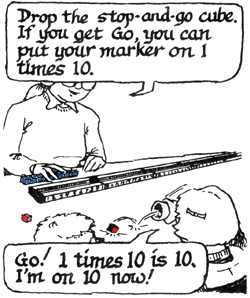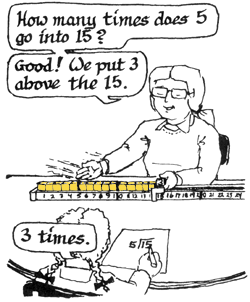# Teaching with Materials

## Dual Board

Teaches place value; addition and subtraction of 2 digit numbers; regrouping; multiplication of 10, 9, 8, and 5.  It is often difficult to demonstrate to students the idea of regrouping. The structure of the Dual Board demonstrates easily that ten ones creates another ten – you physically run out of space for ones – and forces children to regroup them (or exchange them) for a ten-block.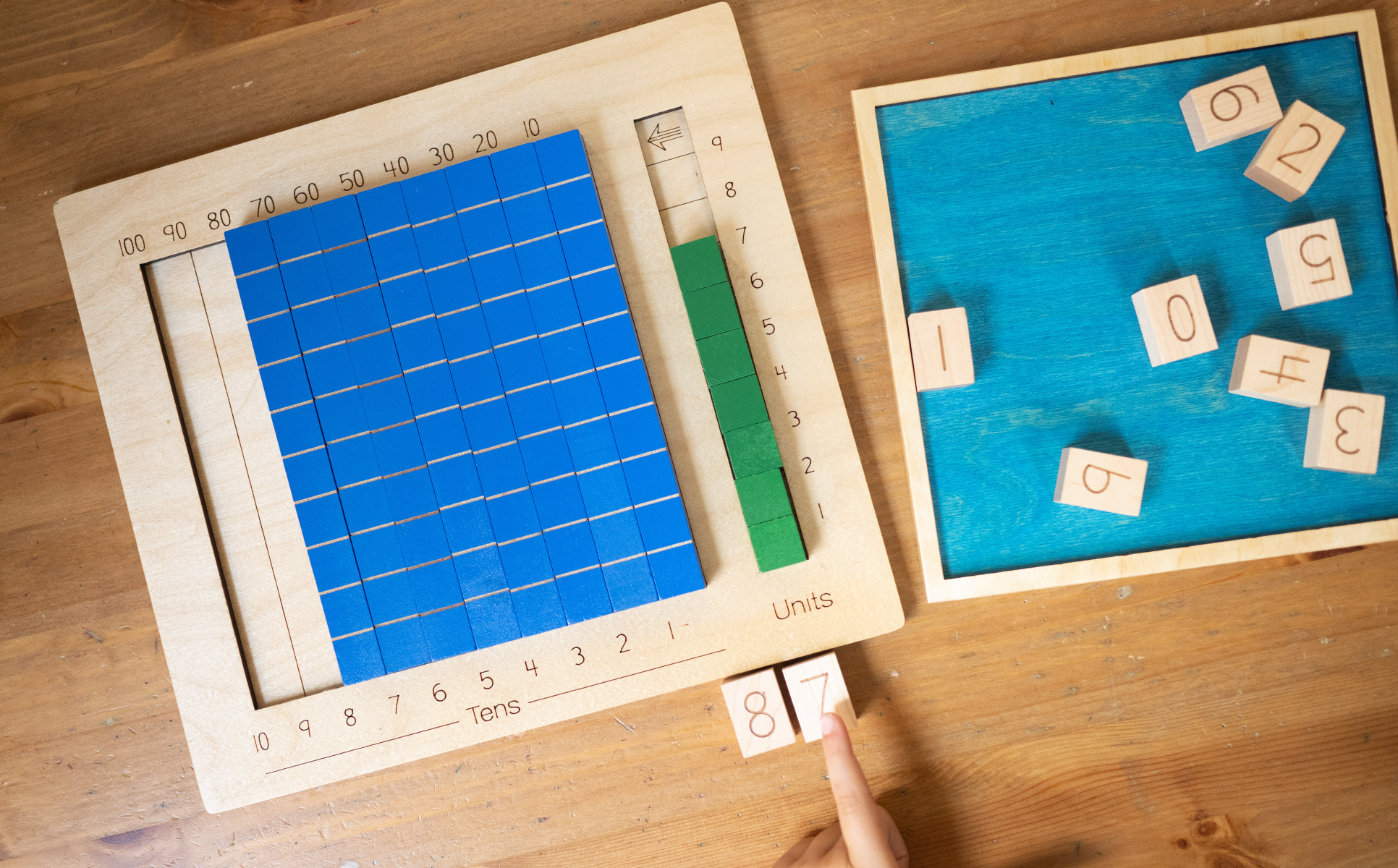The Dual Board is used to teach:

• Place value in 2-digit numbers
• Addition and subtraction of 2-digit numbers
• Regrouping
• Multiplication

## Building 2-Place Numbers in theDual Board

Children build a 2-place number, such as 28, by putting two 10- blocks and eight 1-blocks (single cubes) in the Dual Board. Then they measure the number by placing the blocks in the number track. This gives them both the cardinal and the ordinal aspects of numbers. The Dual Board helps children see the structure of 2-place numbers. The number track helps children see where numbers come in the number sequence.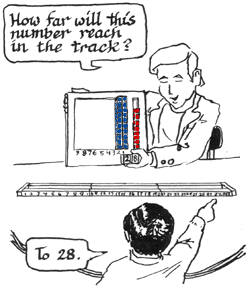## Number Track and Blocks

The Number Track is a concrete representation of the number line, giving children an understanding of the magnitude of “big” numbers. Children build numbers and play games on the number track, developing mental images of those numbers as they develop fluency in all operations.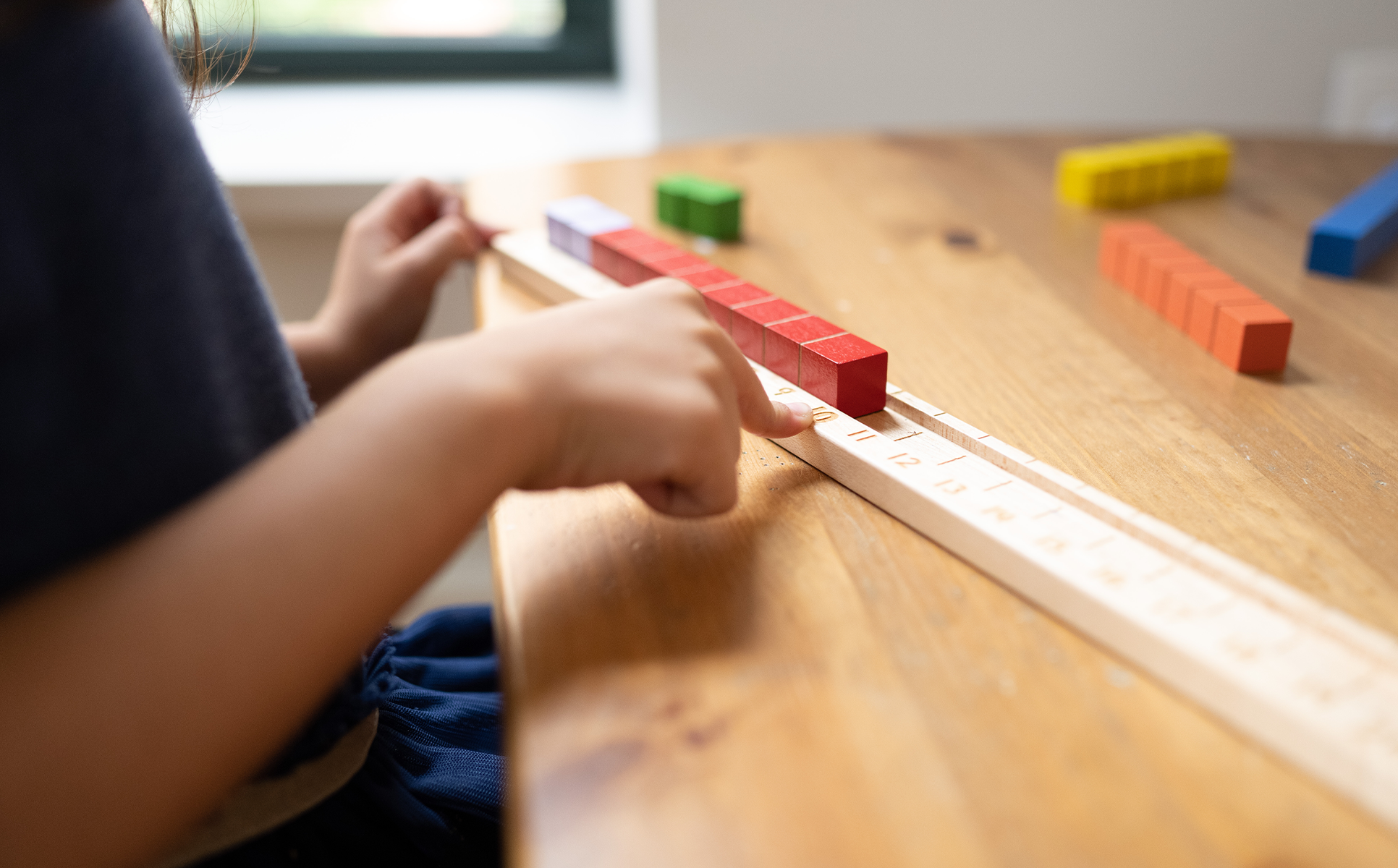The number track is used to demonstrate:

• The place and magnitude of numbers on a number line from 0 to 100
• The decades and the base-ten number system